# Fuzzy matching string comparisons with Dask DataFrames

March 1, 2022

This post explains how to run string comparison algorithms like Levenshtein Distance, Hamming Distance, Jaro Distance, and Match Rating Comparison to compare large datasets of strings stored in Dask DataFrames.

Dask can run fuzzy matching algorithms in parallel so the computations can be scaled to multiple machines and run on large datasets quickly.

Let’s see how to compute the Levenshtein Distance between two string columns in a Dask DataFrame. The Levenshtein Distance is “the minimum number of single-character edits (insertions, deletions or substitutions) required to change one word into the other”. The lower the Levenshtein Distance, the more similar the strings.

We’ll leverage the string similarity algorithms from the jellyfish library to make this computation really easy.

Let’s create a Dask DataFrame with the following two string columns so we have some example data to compute the Levenshtein Distance.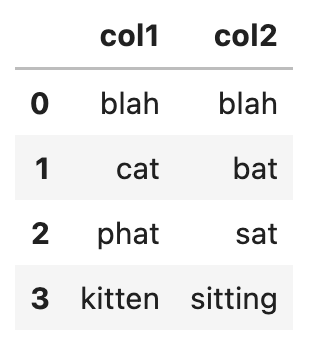Here’s the code to create the Dask DataFrame.

```import dask.dataframe as dd
import jellyfish

import pandas as pd

df = pd.DataFrame(
{
"col1": ["blah", "cat", "phat", "kitten"],
"col2": ["blah", "bat", "sat", "sitting"],
}
)

ddf = dd.from_pandas(df, npartitions=2)```

Now add a column with the Levenshtein Distance to the Dask DataFrame and view the result.

```ddf["col1_col2_lev"] = df.apply(
lambda x: jellyfish.levenshtein_distance(x.col1, x.col2),
axis=1,
)

ddf.compute()```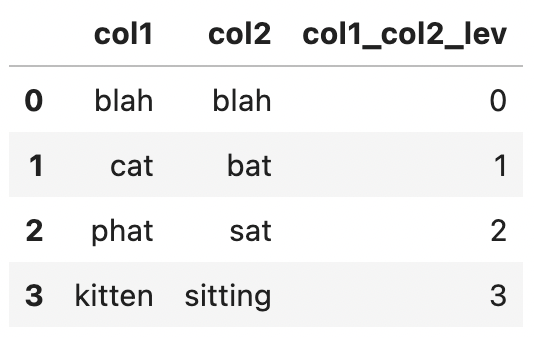The `col1_col2_lev` column contains integer values representing the number of changes that are needed to make the strings equivalent.

## Dask string similarity: Hamming Distance

The Hamming Distance is “the minimum number of substitutions required to change one string into the other, or the minimum number of errors that could have transformed one string into the other”.

Let’s create another DataFrame and compute the Hamming Distance between two string columns. Here’s the data we’ll use.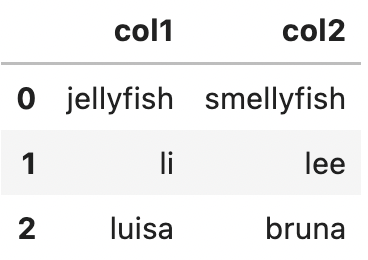Let’s create a Dask DataFrame with this data.

```df = pd.DataFrame(
{
"col1": ["jellyfish", "li", "luisa"],
"col2": ["smellyfish", "lee", "bruna"],
}
)

ddf = dd.from_pandas(df, npartitions=2)```

Now add a col1_col2_hamming column that computes the Hamming Distance between the two string columns.

```ddf["col1_col2_hamming"] = df.apply(
lambda x: jellyfish.hamming_distance(x.col1, x.col2),
axis=1,
)

ddf.compute()```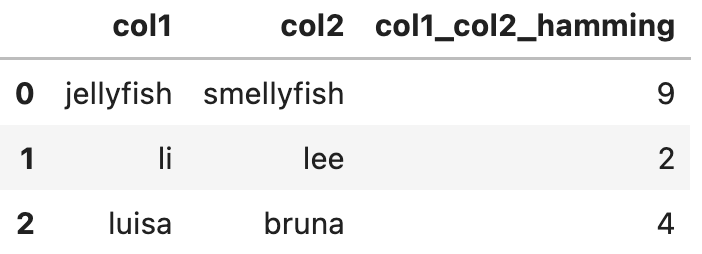## Dask string matching: Jaro Similarity

The Jaro distance is a measure of similarity between two strings. The Jaro distance is a number between 0 and 1 with 0 for strings that aren’t similar at all and 1 for strings that are equal. See this link for the full algorithm.

Let’s use the following data and compute the Jaro Similarity between the two string columns.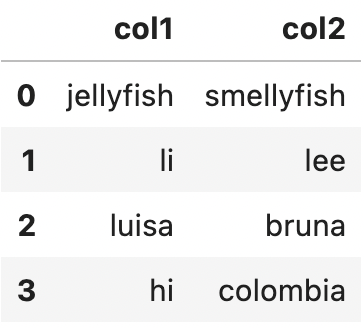Start by creating the Dask DataFrame.

```df = pd.DataFrame(
{
"col1": ["jellyfish", "li", "luisa", "hi"],
"col2": ["smellyfish", "lee", "bruna", "colombia"],
}
)

ddf = dd.from_pandas(df, npartitions=2)```

Now add a `col1_col2_jaro` column to the DataFrame that computes the Jaro Similarity.

```ddf["col1_col2_jaro"] = df.apply(
lambda x: jellyfish.jaro_similarity(x.col1, x.col2),
axis=1,
)

ddf.compute()```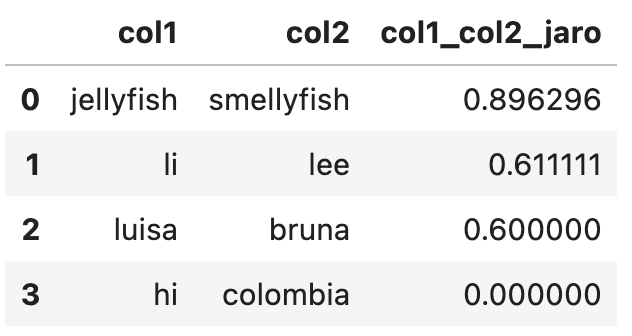The match rating comparison is to test the phonetic equivalence of two strings. It’s a phonetic matching algorithm, which is different from the string similarity algorithms we’ve seen thus far.

Let’s use the following data to compute the match rating comparison value for two string columns.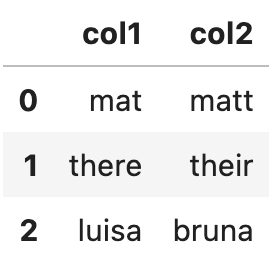Start by creating a Dask DataFrame with the sample data.

```df = pd.DataFrame(
{
"col1": ["mat", "there", "luisa"],
"col2": ["matt", "their", "bruna"],
}
)

ddf = dd.from_pandas(df, npartitions=2)```

Now add a `col1_col2_match_rating` column to the DataFrame with the match rating comparison between `col1` and `col2`.

```ddf["col1_col2_match_rating"] = df.apply(
lambda x: jellyfish.match_rating_comparison(x.col1, x.col2),
axis=1,
)

ddf.compute()```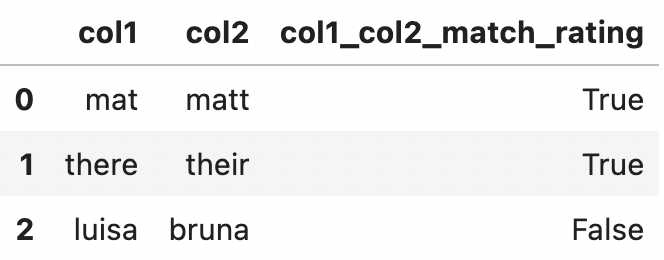Match rating comparisons are a great way to compare strings that sound the same.

## Other string matching algorithms

There are other string matching algorithms, like the ones included in thefuzz.

Dask is great because it makes it easy to run native Python functions in parallel and at scale on large datasets. The jellyfish examples you’ve seen thus far can easily be scaled to run on multiple machines with a powerful computational cluster.

The PyData library ecosystem is extensive and there are a lot of libraries with string matching algorithms relevant for different domains. Dask makes it easy for you to leverage the expansive PyData library ecosystem in your projects.

## Conclusion

You can use Dask and libraries like jellyfish to run string similarity computations on large datasets.

Dask splits up the data and makes it easy to run these computations in parallel, on a single computer or on a cluster of machines. Dask is a great option for fuzzy string comparisons on datasets that are too large for pandas.